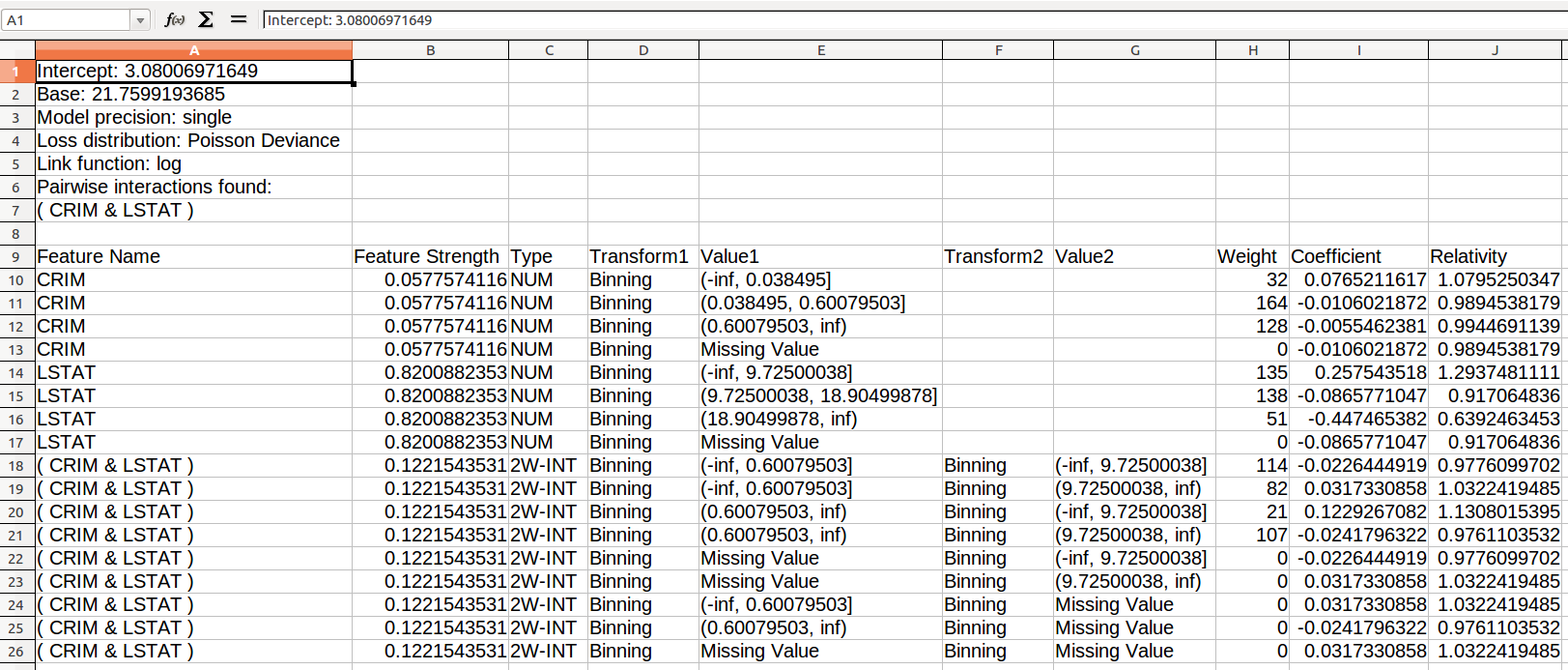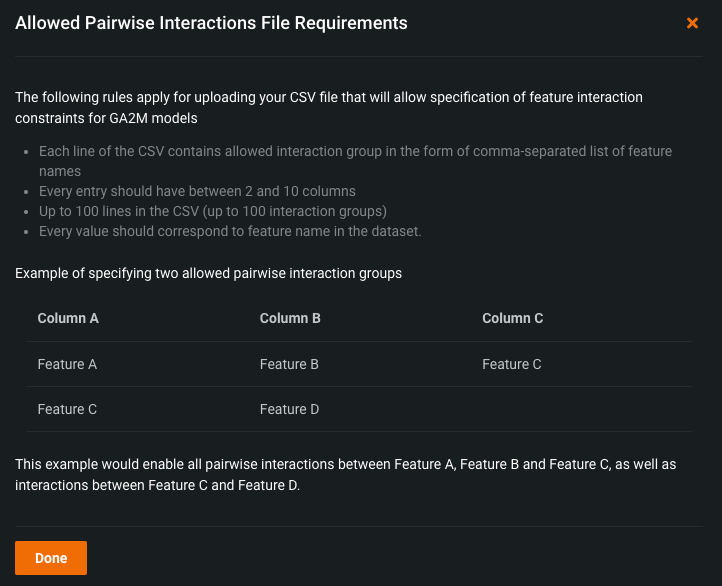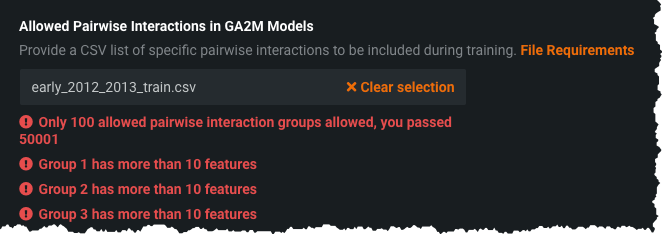Click in-app to access the full platform documentation for your version of DataRobot.

# GA2M output (from Rating Tables)¶

The following section helps to understand the output for Generalized Additive Model (GA2M) models. This output is available as a download from the Rating Tables tab.

When examining the output, note the following:

• Pairwise interactions found by the GA2M model have the following characteristics:

• when there is an interaction of two variables, there is an additional table heading labeled `(Var1 & Var2)`.

• table rows that describe preprocessing and coefficients of pairwise interactions have a Type of `2W-INT`.

• Feature Strength describes the strength of each feature and pairwise interaction. Interaction strength is marginal and doesn't include the main effects strength. The Feature Strength is equal to the weighted average of the absolute value of the centered coefficients.

• Transform1 and Value1 describe the preprocessing of the first variable in the pair; Transform2 and Value2 describe the preprocessing of the second variable in the pair. The coefficient applies to the product of the two values derived from the preprocessing of the two variables.

• Weight is the sum of observations for each row of the table. If the project is using a weight variable, the Weight column is the sum of weights. This can be used to quantify the (weighted) number of observations in the training data that correspond to each bin of numeric feature, each level of categorical feature, or each cell of pairwise interaction.

The following is a sample excerpt from Generalized Additive Model output:In the sample table, the Intercept, Base, Loss distribution, and Link function parameters describe the model in general and not any particular feature. Each row in the table describes a feature and the transformations DataRobot applies to it. To compute the predictions, you can use either the `Coefficient` column or the `Relativity` column. Use the `Coefficient` column if you want the prediction to have the same precision as DataRobot predictions.

For example, assume CRIM value equals 0.9 and LSTAT equals 8.

Using the Coefficient column, read the sample as follows:

For... Coefficient value From line...
Intercept 3.080070 1
Coefficient for CRIM=0.9 -0.005546 12 (bin includes CRIM values 0.60079503 to inf)
Coefficient for LSTAT=8 0.257544 14 (bin includes LSTAT values -inf to 9.72500038)
Get Coefficient for CRIM=0.9 and LSTAT=8 0.122927 20 (bin for Value1, CRIM, equal to 0.9 and Value2, LSTAT equal to 8)

Prediction = exp(3.08006971649 -0.00554623809222501 + 0.257543518013598 + 0.122926708231993) = 31.658089382684512

Using the Relativity column, read the sample as follows:

For... Relativity value From line...
Base 21.7599 2
Relativity for CRIM=0.9 -0.9945 12 (bin includes CRIM values 0.60079503 to inf)
Coefficient for LSTAT=8 1.2937 14 (bin includes LSTAT values -inf to 9.72500038)
Get Coefficient for CRIM=0.9 and LSTAT=8 1.1308 20 (bin for Value1, CRIM, equal to 0.9 and Value2, LSTAT equal to 8)

Prediction = 21.7599193685 * 0.994469113891232 * 1.29374811110316 * 1.13080153946617 = 31.65808938265751

If the main model uses a two-stage modeling process (Frequency-Severity Generalized Additive Model, for example), two additional columns—`Frequency_Coefficient` and `Severity_Coefficient`—provide the coefficients of each stage.

## Allowed pairwise interactions in GA2M¶

You can choose to control which pairwise interactions are included in Generalized Additive Models' output (available in the Rating Tables tab) instead of using every interaction or none of them. This allows you to specify which interactions are permitted to interact during the training of a GA2M model in cases where there are certain features that are not permitted to interact due to regulatory constraints.

Note

Note that specified pairwise interactions are not guaranteed to appear in a model's output. Only the interactions that add signal to a model according to the algorithm will be featured in the output. For example, if you specify an interaction group of features A, B, and C, then AxB, BxC, and AxC are the interactions considered during model training. If only AxB adds signal to the model, then only AxB is included in the model's output (excluding BxC and AxC).

To specify the allowed pairwise interactions for a model, navigate to Advanced options on the Start screen. Under the Feature Constraints tab, you can configure your allowed pairwise interactions.You must provide a CSV file that specifies the pairwise interactions you want to include. Click the File Requirements link (1) for more information about the format of the CSV file. Specifically, it addresses the limitations and format of CSVs used for this purpose. It also includes an example table showing how to structure your CSV in a case that specifies two allowed pairwise interaction groups.Apply the required formatting and limitations to the CSV, and then click Browse (2) to upload it (or drag and drop). DataRobot then begins validating the CSV to ensure it matches the file requirements, and indicates any formatting errors with a message:After successfully uploading a CSV with proper formatting, you can begin training GA2M models. When the models are built, examine their output in the Rating Tables tab. The output only indicates the pairwise interactions that you specified.

## Define transformations for GA2M¶

The following sections describe the routines DataRobot uses to reproduce predictions from a GAM.

### One-hot encoding¶

Name: One-hot Value: string, or `Missing value`, or `Other categories` Value example: 'MA' Value example: Missing value

One-Hot (or dummy-variable) transformation of categorical features:

• If `value` is a string then derived feature will contain 1.0 whenever the original feature equals `value`.

• If value of the original feature is missing then "Binning" transformation with "Missing value" is equal to 1.0.

• If `value` is "Other categories" then derived feature will contain 1.0 when the original feature doesn't match any of the above.

### Dummy encoding¶

Name: Dummy Value: string Value example: 'MA'

Derived feature will contain 1.0 whenever the original feature equals `value`.

### 1-Dummy encoding¶

Name: 1-Dummy Value: string Value example: 'NOT MA'

Derived feature will contain 1.0 whenever the original feature is different from `value` without the 4 characters 'NOT '.

### Binning¶

Name: Binning Value: (a, b], or `Missing value` Value example: (-inf, 12.5] Value example: (12.5, 25] Value example: (25, inf) Value example: Missing value

Transform numerical variables into non-uniform bins.

The boundary of each bin is defined by the two numbers specified in `value`. Derived feature will equal to 1.0 if the original value `x` is within given interval:

``````a < x <= b
``````

If `value` of the original feature is missing, then "Binning" transformation with "Missing value" is equal to 1.0.

Updated November 17, 2021